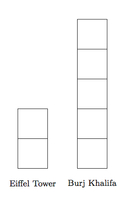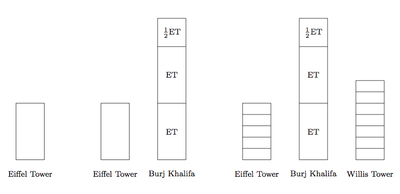# Comparing Heights of Buildings

Alignments to Content Standards: 5.NF.B.5 5.NF.B.6

The Burj Khalifa (Dubai) is about 2$\frac{1}{2}$ times as tall as the Eiffel Tower (Paris) . The Eiffel Tower is about $\frac{5}{7}$ as tall as the Willis Tower (Chicago).

1. Which of these buildings is the tallest? Which is the shortest? Explain.
2. Draw pictures to illustrate.

## IM Commentary

The goal of this task is to compare three quantities using the notion of multiplication as scaling. Students will recognize (5.NF.B.5) that the Burj Khalifa is taller than the Eiffel tower and that the Eiffel Tower is shorter than the Willis Tower using the size of the given multiplicative scalars. More work is necessary, however, to compare the Willis Tower to the Burj Khalifa because these heights are only given in reference to the height of the Eiffel Tower. Students can compare these two heights either by reasoning about the size of the two given scalar multiples or using pictures as in part (b). For a follow-up question, the teacher may wish to ask students how many times taller the Burj Khalifa is than the Willis Tower: the pictures in part (b) will help with this but students will need to break both the Willis Tower and the Burj Khalifa into equal sized parts of the Eiffel Tower (tenths) to answer the question.

The Burj Khalifa is currently the world's tallest building. Interestingly, both the Eiffel Tower and the Willis Tower were the tallest building in the world at the time of their construction. Much more information about these buildings and others can be found here: http://en.wikipedia.org/wiki/List_of_tallest_buildings_and_structures_in_the_world. This task was developed with the assistance of a group of teachers from Washington and Illinois in connection with an SBAC digital library project. One goal of this work was to highlight different aspects of the ratio and proportion progression. Multiplication as scaling (5.NF.5) provides important experience viewing one quantity as a multiple of another, laying the foundation for ratios.

This task was adapted from https://www.illustrativemathematics.org/illustrations/143 in order to provide a context relevant for the Chicago audience for this work. In contrast to the task on which it was based, students must provide the picture in this case, giving the alignment with 5.NF.B.6.

This task can be used as formative assessment at the very beginning of a unit focusing on 6.RP. The skill assessed in this task, comparing two quantities using multiplication or a scale factor, is fundamental to all reasoning about ratios: in fact, although the statement of the problem can be viewed as multiplication, it is also an example of a ratio because none of the heights of the buildings are provided. If students have difficulty with this task, teachers may wish to review this material briefly. In addition, this example could be revisited as students learn more about the language of ratios. For example, in the statement of the problem, ''The Burj Khalifa is 2$\frac{1}{2}$ times as tall as the Eiffel Tower,'' this is an example of a unit rate. The Eiffel Tower is being taken as the unit of measure. In the comparison of the Eiffel Tower with the Willis Tower, however, the Willis Tower is being taken as the unit. With ratios, the relationship between the height of the Burj Khalifa to the Eiffel Tower might be expressed as 5:2, providing a link with the improper fraction form, $\frac{5}{2}$, of 2$\frac{1}{2}$. Finally, the pictures requested in part (b) are good examples of strip diagrams, an important and basic technique for representing ratios.

This task was written as part of a collaborative project between Illustrative Mathematics, the Smarter Balanced Digital Library, the Teaching Channel, and Desmos.

## Solution

1. Since 2$\frac{1}{2}$ is more than 1, the Burj Khalifa is taller than the Eiffel Tower: in fact it is more than twice as tall. Since $\frac{5}{7}$ is less than $\frac{7}{7}$ or 1, this means that the Eiffel Tower is shorter than the Willis Tower. So the Eiffel Tower is the shortest of the three buildings.

To compare the Burj Khalifa to the Willis Tower, we can see that $\frac{5}{7}$ is more than a half so the Eiffel Tower is more than half as tall as the Willis Tower. Since the Eiffel Tower is less than half as tall as the Burj Khalifa this means that the Burj Khalifa is the tallest of the three buildings.
2. The Burj Khalifa is 2$\frac{1}{2}$ or $\frac{5}{2}$ times as tall as the Eiffel Tower.  This means that if we take half of the Eiffel Tower as a unit then the Eiffel Tower is two of these units while the Burj Khalifa is 5 as shown below:The Eiffel Tower is $\frac{5}{7}$ as tall as the Willis Tower so here we take $\frac{1}{7}$ of the Willis Tower as our unit. We know that 5 of these units will model the height of the Eiffel Tower while 7 of them gives the height of the Willis Tower:Below are pictures showing how to build a model of all three building heights together:The first picture shows just the Eiffel Tower. The second picture shows the Eiffel Tower and the Burj Khalifa, with 2$\frac{1}{2}$ Eiffel Towers in the Burj Khalifa. The final picture has all three buildings, showing that the Willis Tower is $\frac{7}{5}$ times as tall as the Eiffel Tower.